Stress for Thick Walled Cylinders using Lamé’s Equations

Thick Walled Cylinder Stress Calculator

Summary

Three of the primary mechanical stresses (not to be confused with ‘principle stresses’) that can be applied to a cylindrically shaped object are:

• Hoop Stress
• Axial Stress

If the object/vessel has walls with a thickness greater than one-tenth of the overall diameter, then these objects can be assumed to be ‘thick-walled’. The general equations to calculate the stresses are:

• Hoop Stress,

(1)(2)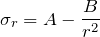From a thick-walled cylinder, we get the boundary conditions: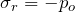at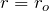andat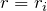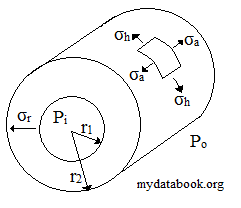Applying these boundary conditions to the above simultaneous equations gives us the following equations for the constants A & B:

(3)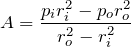(4)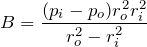Finally, solving the general equations with A & B gives Lamé’s equations:

• Hoop Stress,

(5)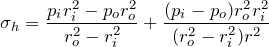(6)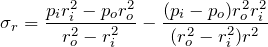The axial stress for a closed-ended cylinder is calculated by means of the equilibrium, which reduces to:

• Axial Stress,

(7)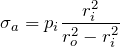Thick Wall Cylinder Hoop Stress Calculator

 Calculate the hoop stress in a thick-walled cylinder: Formula: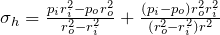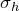= Hoop stress Pi = internal pressure Po = external pressure ri = internal radius ro = external radius r = radius at point of interest (usually ri or ro) Internal Pressure Pa External Pressure Pa Internal Radius m External Radius m Radius at Point of Interest m Result: Pa

Thick Wall Cylinder Radial Stress Calculator

 Calculate the radial stress in a thick-walled cylinder: Formula: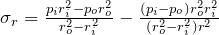= radial stress Pi = internal pressure Po = external pressure ri = internal radius ro = external radius r = radius at point of interest (usually ri or ro) Internal Pressure Pa External Pressure Pa Internal Radius m External Radius m Radius at Point of Interest m Result: Pa

Thick Wall Cylinder Axial Stress Calculator

 Calculate the axial stress in a closed-ended thick-walled cylinder: Formula: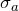= axial stress Pi = internal pressure ri = internal radius ro = external radius Internal Pressure Pa Internal Radius m External Radius m Result: Pa